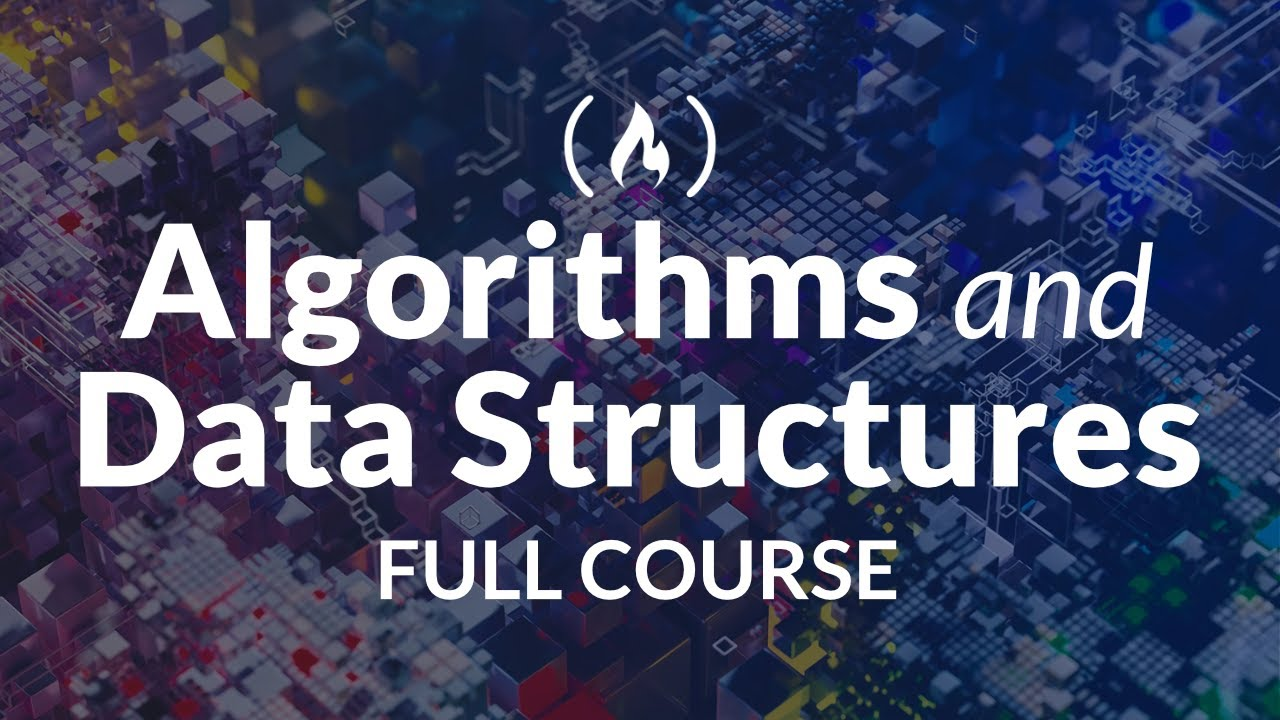# Algorithms and Data Structures - Full Course for Beginners from TreehouseIn this course you will learn about algorithms and data structures, two of the fundamental topics in computer science. There are three main parts to this course: algorithms, data structures, and a deep dive into sorting and searching algorithms.

In this course you will learn about algorithms and data structures, two of the fundamental topics in computer science. There are three main parts to this course: algorithms, data structures, and a deep dive into sorting and searching algorithms.

By the end, you will understand what algorithms and data structures are, how they are measured and evaluated, and how they are used to solve problems.

This course was developed by Treehouse teachers Pasan Premaratne and Jay McGavren. Join Treehouse to access more great courses: https://join.teamtreehouse.com/treehouse-new-student/

⭐️ Course Contents ⭐️
⌨️ (0:00:00) Introduction to Algorithms
⌨️ (1:57:44) Introduction to Data Structures
⌨️ (4:11:02) Algorithms: Sorting and Searching